# SPSS tutorials

BASICS DATA ANALYSIS T-TEST ANOVA CHI-SQUARE TEST

# SPSS Independent Samples T-Test Tutorial

## Null Hypothesis

The null hypothesis for an independent samples t test is that two populations have equal means on some metric variable.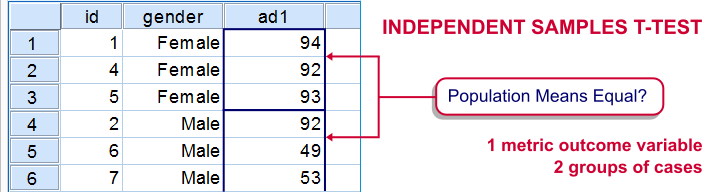For example, do men spend the same amount of money on clothing as women? We can't reasonably ask the entire population of men and women how much they spend. So we'll draw a sample of men and women. These samples are independent because they don't overlap: everybody is either man or woman, never both.
Now, sample outcomes tend to differ a bit from population figures. So if the average amount spent is precisely equal for all men and women, we'll probably still see slightly different means between our samples. However, very different sample means suggest that the population means weren't equal after all. A t test tells us if a sample difference is big enough to draw this conclusion.

## SPSS Independent T Test Example

A scientist wants to know if children from divorced parents score differently on some psychological tests than children from non divorced parents. The data collected are in divorced.sav, part of which is shown below.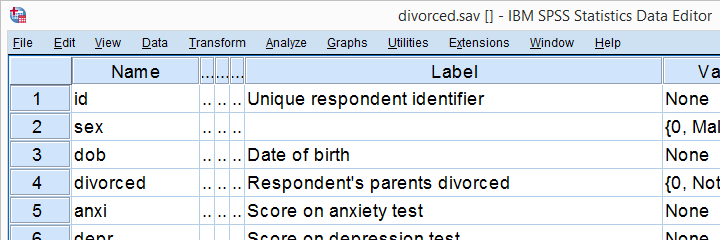The last 4 variables in our data file hold our test scores. For each variable, we'll use a t test to evaluate if the mean scores are different between our 2 groups of children.

## Independent Samples T Test - Assumptions

Conclusions from an independent samples t test can be trusted if the following assumptions are met:

1. Independent observations. This often holds if each case in SPSS represents a different person or other statistical unit. This seems to hold for our data.
2. Normality: the dependent variable must follow a normal distribution in the population. This is only needed for samples smaller than some 25 units. We'll see the actual samples sizes used for our t test after running it so we won't bother about normality until then.
3. Homogeneity: the standard deviation of our dependent variable must be equal in both populations. We only need this assumption if our sample sizes are (sharply) unequal.
SPSS tests if this holds when we run our t test. If it doesn't, we can still report corrected test results.

If these assumptions are badly violated, you could consider using a Mann-Whitney test instead of a t test. This is suitable for ordinal variables as well.

## Quick Data Check

The data at hand have been prepared and are good to go. However, if you run a t test on other data, you should at least inspect some histograms of your dependent variable(s). Make sure their distributions look plausible. If they contain any extreme values, specify them as user missing values.

## Running an Independent Samples T Test in SPSS

Running an independent samples t test in SPSS is pretty straightforward. The screenshots below walk you through.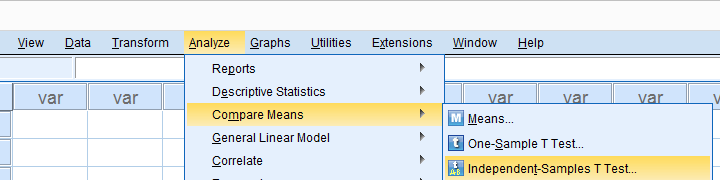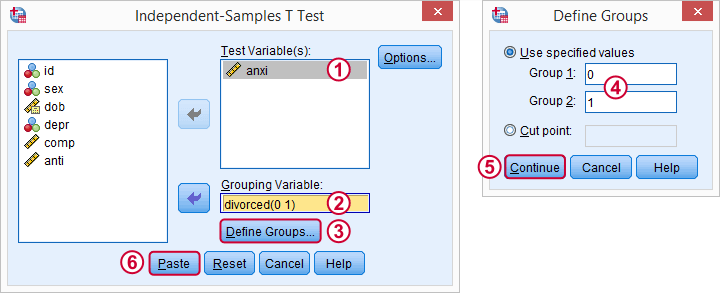We'll first test anxi and make sure we understand the output. We'll get to the other 3 dependent variables later.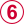Clicking creates the syntax below. Let's run it.

## SPSS Independent Samples T Test Syntax

*Independent-samples t-test syntax for anxi by divorced.

T-TEST GROUPS=divorced(0 1)
/MISSING=ANALYSIS
/VARIABLES=anxi
/CRITERIA=CI(.95).

## SPSS Output for an Independent Samples T Test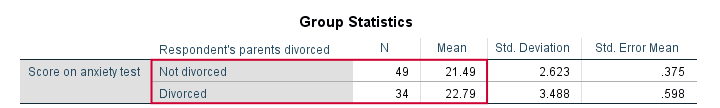We first take a look at Group Statistics. First off, note that there's only a small difference between our sample means. Children from divorced parents have an average anxiety score of 22.8 whereas the other children score 21.5.
Second, note that the sample sizes used for our t test are 49 and 34. Since both are larger than 25, we don't need to bother about the normality assumption.
If you encounter smaller sample sizes while analyzing other data, you may check for normality by inspecting histograms or running a Kolmogorov-Smirnov test.We don't recommend this test because it has low power in small samples. Since it still seems to convince a lot of people, you may consider using it anyway.

## Independent Samples T Test Output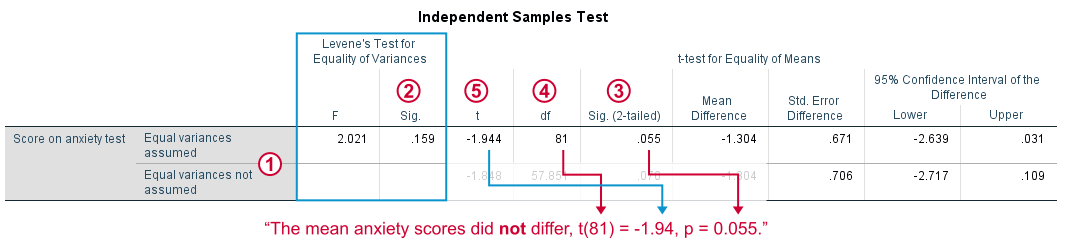Note that we have two lines of t test results: equal variances assumed and equal variances not assumed. So which line should we report? Well, this depends on Levene's test for equal variances which tests the aforementioned homogeneity assumption.As a rule of thumb, if Sig. > 0.05, we conclude that the assumption of equal variances holds. Since Sig. = 0.159 here, we report the first line of t test results, denoted as equal variances assumed.If Sig. (2-tailed) > 0.05, we usually conclude that our population means are equal. “Sig.” is called a significance level (or just “p”) in reports. P indicates how likely our sample result is if our population means are really equal. In our case, p = 0.055 (a 5.5% probability) and that's not unlikely enough for rejecting our null hypothesis.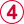df (degrees of freedom) is not really interesting but we'll report it anyway. The same goes fort, our test statistic.

## What About the Other Variables?

Right, let's now analyze all 4 test scores. We can do so by reopening the t test dialog from the menu (tip: try the dialog recall tool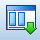here). Alternatively, just add the variable names to the previously used syntax.

*Independent-samples t-test syntax for anxi by divorced.

T-TEST GROUPS=divorced(0 1)
/MISSING=ANALYSIS
/VARIABLES=anxi depr comp anti
/CRITERIA=CI(.95).

## Result

At this point you should be able to draw the right conclusions. The null hypothesis of equal population means is rejected only for our last two variables: compulsive behavior, t(81) = -3.16, p = 0.002 and antisocial behavior, t(51) = -8.79, p = 0.000.
The figure below shows how we first inspect Sig. for Levene's test and then choose which t test results we report.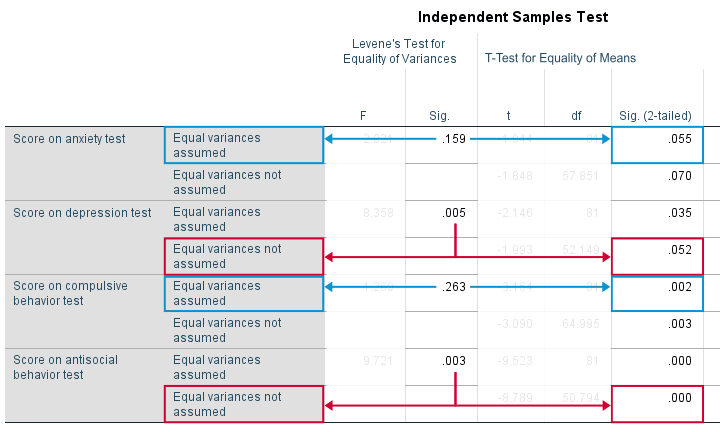## Reporting an Independent Samples T Test

First off, report means and standard deviations for both groups. Perhaps include sample sizes as well: for multiple tests, these may vary due to missing values. I like reporting such descriptive statistics in a simple overview table as shown below.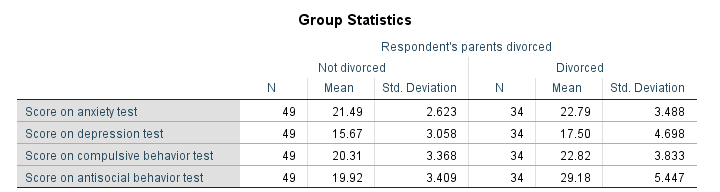You could add some columns to this table holding df, t and p for each test (p is denoted as “Sig. (2-tailed)” in SPSS).

Alternatively, report each t test result as “Children from divorced parents scored higher on compulsive behavior than other children, t(81) = -3.16, p = 0.002.”

# Let me know what you think!

*Required field. Your comment will show up after approval from a moderator.

# This tutorial has 64 comments

•### By EH on March 7th, 2018

Very helpful description! I am wondering if it's possible to do a post-hoc analysis (such as least significant difference) with an independent samples t-test? I know one can always do a Bonferroni (even manually), but Bonferroni is super conservative!

•### By Dr Shine David on February 18th, 2018

very nice and simply explained.

•### By Ruben Geert van den Berg on February 13th, 2018

Oh, I forgot a very recent -and popular- one: Statistical Significance – What Does It Really Mean?. That should get you started fast with basically all statistical tests.

•### By Ruben Geert van den Berg on February 13th, 2018

Perhaps reading up on some very basic statistics tutorials will help? I suggest you take a look at

Sampling Distribution - What is It?

Standard Deviation - What Is It?

and perhaps

How to Run Levene’s Test in SPSS?

A more detailed tutorial on just this t-test will come up as well, probably in some 3-4 weeks. It'll be published on our facebook page.

Hope that helps!

•### By Ahmad Abdallah on February 13th, 2018

Frankly, due to the fact that this topic is brand new to me and never read it or heard about it, I am facing major difficulties in understanding it. The abbreviations used are not familiar to me.

I really wish if you have a more detailed explanation to